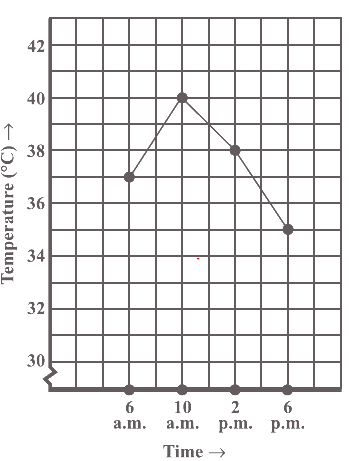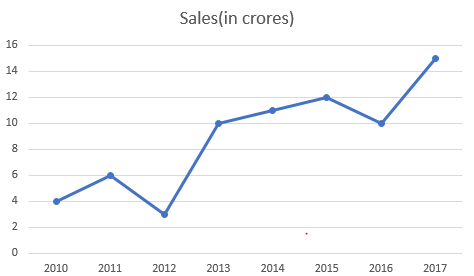# introduction to graphs class 8 extra questions

In this page we have introduction to graphs class 8 extra questions . Hope you like them and do not forget to like , social share and comment at the end of the page.
Question 1
The number of electric bulbs manufactured by a factory during five consecutive years is given below.
 Years 2006 2007 2007 2008 2009 Number of Bulbs(lakh) 2 8.5 7 5 1
Draw the Line graph representing the above data.
Question 2
Plot the points on a graph: P(4, 9) Q(6, 0) R(7, 7) S(2, 4)

Question 3
The number of students in Sandeep coaching institute during last 8 years is given below. Represent this data on the Line graph
 Years 2001 2002 2003 2004 2005 2006 2007 Number of Students 100 120 95 130 85 160 100

Question 4
True and False Statement
(a) (5,0) lies on x-axis
(b) (0,1) lies on x-axis
(c) (0,0) is the origin
(d) (4,4) is equal distance from both the x-axis and y-axis
Question 5
The number of trees planted by Government in different years is given below.
 Year 2010 2011 2012 2013 2014 2015 Number of Trees to be Planted 150 220 350 400 300 380
Draw the line graph to represent the data.

Question 6.(a) what time temperature was maximum
(b) What is the temperature at 6 PM
(c) What is the temperature difference between time 6:00 AM and 6:00 PM

Question 7
Draw the points (1, 4) and (4, 1). Do they represent the same point?
Question 8.What were the sales in
(i) 2012
(ii) 2016
(iii)2007
(iv) 2014
(b) Compute the difference between the sales in 2012 and 2016.
(c) In which year was there the greatest difference between the sales as compared to its previous year?
Question 9
The average monthly attendance of a class is given.
 Month July Aug Sep Oct Nov Dec Jan Feb Attendance 40 45 30 50 55 30 35 40
Draw the bar graph of the data given.

Question 10.
Draw the coordinates (2,2) , (2,4) and (4,2) And join these to form the triangle.

## Summary

This introduction to graphs class 8 extra questions is prepared keeping in mind the latest syllabus of CBSE . This has been designed in a way to improve the academic performance of the students. If you find mistakes , please do provide the feedback on the mail. You can download this also using the below link